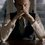# Product of all such expressions is a positive integer

If $P(n)$ denotes the product of all the numbers of the form $\pm \sqrt{1} \pm \sqrt{2} \pm \sqrt{3}\pm \cdots \pm \sqrt{n}$ where $\pm$ are chosen arbitrarily.

Then prove that $P(n)$ is a positive integer $\forall n \geq 2$.

(Can we find that positive integer?)Note by Kartik Sharma
4 years, 6 months ago

This discussion board is a place to discuss our Daily Challenges and the math and science related to those challenges. Explanations are more than just a solution — they should explain the steps and thinking strategies that you used to obtain the solution. Comments should further the discussion of math and science.

When posting on Brilliant:

• Use the emojis to react to an explanation, whether you're congratulating a job well done , or just really confused .
• Ask specific questions about the challenge or the steps in somebody's explanation. Well-posed questions can add a lot to the discussion, but posting "I don't understand!" doesn't help anyone.
• Try to contribute something new to the discussion, whether it is an extension, generalization or other idea related to the challenge.

MarkdownAppears as
*italics* or _italics_ italics
**bold** or __bold__ bold
- bulleted- list
• bulleted
• list
1. numbered2. list
1. numbered
2. list
Note: you must add a full line of space before and after lists for them to show up correctly
paragraph 1paragraph 2

paragraph 1

paragraph 2

[example link](https://brilliant.org)example link
> This is a quote
This is a quote
    # I indented these lines
# 4 spaces, and now they show
# up as a code block.

print "hello world"
# I indented these lines
# 4 spaces, and now they show
# up as a code block.

print "hello world"
MathAppears as
Remember to wrap math in $$ ... $$ or $ ... $ to ensure proper formatting.
2 \times 3 $2 \times 3$
2^{34} $2^{34}$
a_{i-1} $a_{i-1}$
\frac{2}{3} $\frac{2}{3}$
\sqrt{2} $\sqrt{2}$
\sum_{i=1}^3 $\sum_{i=1}^3$
\sin \theta $\sin \theta$
\boxed{123} $\boxed{123}$

Sort by:

Here's my attempt: Call this set $S$. The set $S$ has $2^{n}$ members. No such member can be zero. There is a one-one correspondence between a positive member $x \in S$ and $-x \in S$ (note that both $x$ and $-x$ will belong to this set and this is seen by switching all the signs). Their product is $-x^2 < 0$. And the number of such pairs is $2^n/2 = 2^{n-1}$. For $n \ge 2$, this is an even natural number. And the product of an even number of pairs, where the product of each pair is negative, is of course, positive.

- 4 years, 2 months ago

My try is as follows.

Let $P_{n-1}(x) = a_{2^{n-1}}x^{2^{n-1}} + a_{2^{n-1}-1}x^{2^{n-1}-1} + \cdots + a_1 x^1 + a_0$

be the polynomial having roots as numbers of the form $\pm \sqrt{1} \pm \sqrt{2} \pm \sqrt{3} \pm \cdots \pm \sqrt{n-1}$

Then, $P_n(x)$ has roots as $\text{Root of} P_{n-1}(x) \pm \sqrt{n}$. Thus we can transform it as-

$P_n(x) = (a_{2^{n-1}}{(x-\sqrt{n})}^{2^{n-1}} + a_{2^{n-1}-1}{(x-\sqrt{n})}^{2^{n-1}-1} + \cdots + a_1 {(x-\sqrt{n})}^1 + a_0)(a_{2^{n-1}}{(x+\sqrt{n})}^{2^{n-1}} + a_{2^{n-1}-1}{(x+\sqrt{n})}^{2^{n-1}-1} + \cdots + a_1 {(x+\sqrt{n})}^1 + a_0)$

Proposition: $P_n(x) \text{is a polynomial having integer coefficients}$

We prove this using induction.

Base Case - $n = 1 : x^2 -1 = 0$

Assuming for $n-1$, let's try for $n$,

$\displaystyle [x^k]{P_n(x)} = \sum_{b + c = k}{\left(\sum_{m=0}^{2^{n-1}}{\binom{m}{b} a_m {(\sqrt{n})}^{m-b}}\right)\left(\sum_{t=0}^{2^{n-1}}{\binom{t}{c} a_t {(-\sqrt{n})}^{t-c}}\right)}$

(Oh, that is scary, but it is not required).

We can get a $\sqrt{n}$ term left if $m-b = \text{Odd}, t-c = \text{Even}$ or $t-c = \text{Odd}, m-b = \text{Even}$

Now, an irrational term or a non-integer term(in this case), will be left if either

$m-b = \text{Odd}, t -c = \text{Even}$

or

$m-b = \text{Even}, t -c = \text{Odd}$.

And now if there is a case like $(m-b, t-c) = (1,2)$, then there will also be a case like $(m-b,t-c) = (2,1)$. Note that this counter-case will not be there if $m-b = t-c$ but then their parity is same too, so they will not be considered.

Since by interchanging $(m,b) \rightarrow (t,c)$, we change the coefficient of $n^{(m+t-b-c)/2}$from

$\binom{m}{b}\binom{t}{c}a_m a_t \rightarrow -\binom{m}{b}\binom{t}{c}a_m a_t$

all the numbers with fractional powers cancel out and only integers remain.

By observing the general polynomial recurrence we can see that leading coefficient is given by

$a_n = a_{n-1}^2; a_1 = 1 \implies a_n = 1$

And we have just proven that all the other coefficients are integers.

Therefore, all symmetric products of numbers of the form $\pm \sqrt{1} \pm \sqrt{2} \pm \cdots \pm \sqrt{n}$ is an integer.

- 4 years, 6 months ago

It's slightly hard to follow exactly what you're doing, but I believe you have the right idea.

In the final line, I believe you mean "product" instead of "sum".

Staff - 4 years, 5 months ago

Oops, yeah. Fixed it! What part exactly is not clear? Maybe the formatting isn't good.

- 4 years, 5 months ago

1. Explaining the roots transformation better. (I agree that what you're doing works, but you should be explaining it for others to easily understand why what you're doing works)

2. Note that the induction should start with $n = 2$.

3. Note that you want to show the product is positive, which has been ignored. In particular, looking at $n = 1$, you should realize that the product is $-1$ (which is why we have to start with $n = 2$).

4. Justifying the formula for $[ x^k ] P_n(x)$. At least give a verbal description of how that is arrived at.

Staff - 4 years, 5 months ago

Hey,great solution.BTW you are in which class?

- 4 years, 6 months ago

Class XII.

- 4 years, 6 months ago

You have immense amount of knowledge as compared to a 12th grader!!!!

- 4 years, 6 months ago

I just have a good amount of time to devote to science and mathematics.

- 4 years, 6 months ago